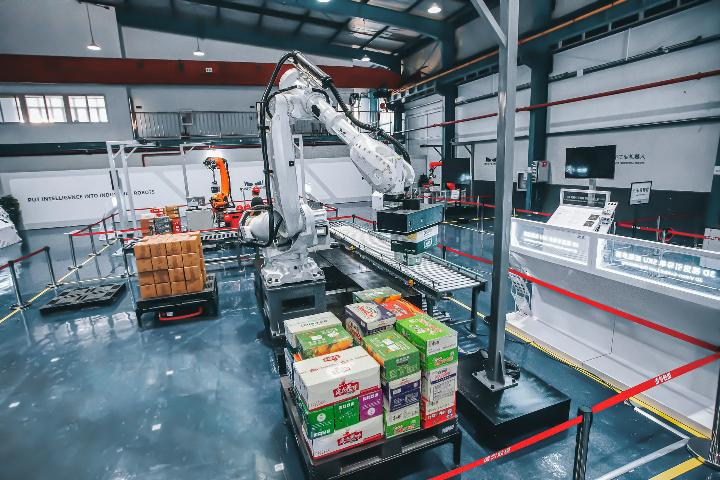# How to Calculate Total Manufacturing Cost: A Step-by-Step Guide

How to calculate total manufacturing cost? Manufacturing businesses rely on accurate cost calculation to ensure profitability and sustainable growth. In this article by Viindoo, we will explore the steps involved in calculating total manufacturing cost. By following these steps, businesses can make informed decisions and optimize their production process to reduce costs and maximize profits. From raw materials to overhead expenses, we will cover all the factors that contribute to total manufacturing cost. Stay tuned to learn the secrets of calculating manufacturing cost like a pro.

## What is the manufacturing cost?

Manufacturing cost refers to the total cost of producing a product, which includes all direct and indirect expenses associated with the production process. This includes the cost of raw materials, direct labor costs (wages, salaries, and benefits of workers involved in the production process), and indirect manufacturing overhead costs (such as rent, utilities, depreciation of machinery, and maintenance costs).

How to calculate total manufacturing cost per unit is crucial for businesses to determine the total expenses incurred in producing their products, and to determine the selling price of the products. By understanding their manufacturing costs, businesses can identify areas for cost reduction and improve their profitability.What is the manufacturing cost?

## How to Calculate Total Manufacturing Cost

### The formula to calculate manufacturing cost per unit

Manufacturing cost per unit = (raw materials cost + direct labor cost + manufacturing overhead cost) / number of units produced

Where:

• Raw materials cost: The cost of all the materials used in the production process.
Direct labor cost: The cost of wages, salaries, and benefits paid to workers directly involved in the production process.
• Manufacturing overhead cost: The cost of all the indirect costs associated with the production process, such as rent, utilities, and maintenance.
• Number of units produced: The total number of units produced in a given period of time.

It is important to note that the formula can be modified or adjusted depending on the specific needs of the manufacturer. Additionally, it is crucial to accurately track and record cost accounting for manufacturing process to ensure the accuracy of the calculation.How to calculate total manufacturing cost per unit

### 5 steps - how to calculate total direct manufacturing cost

• Step 1. Identify the direct materials cost: Determine the cost of the raw materials used in the production process. You can use the following formula:
Cost of raw materials = Beginning inventory + Purchases added - Ending inventory
• Step 2. Calculate the direct labor cost: Determine the wages, salaries, and benefits paid to workers directly involved in the production process.
• Step 3. Determine the manufacturing overhead cost: How to calculate total manufacturing overhead cost? Identify and calculate all indirect costs associated with the production process, such as rent, utilities, depreciation of machinery, and maintenance costs.
• Step 4. Add up all the costs: Add the direct materials cost, direct labor cost, and manufacturing overhead cost to determine the total manufacturing cost.
• Step 5. Determine the cost per unit: Divide the total manufacturing cost by the number of units produced to determine the cost per unit.How to calculate estimated total manufacturing overhead cost

### Example of how to calculate the total manufacturing cost

Let's say a company manufactures 1,000 units of a product in a month, and the following costs were incurred:

• Direct materials cost: \$5,000
• Direct labor cost: \$3,000

To calculate the manufacturing cost per unit, we would add the direct materials cost, direct labor cost, and manufacturing overhead cost, then divide by the number of units produced:

• Manufacturing cost per unit = (\$5,000 + \$3,000 + \$2,000) / 1,000 units
• Manufacturing cost per unit = \$10 per unit

So, the manufacturing cost per unit is \$10. This means that the company incurs a cost of \$10 for every unit produced. By knowing the manufacturing cost per unit, the company can set a selling price that covers the cost of production and generates a profit.Example of how to calculate the total manufacturing cost

This article provides readers with the answer to the question “how to calculate total manufacturing cost". Calculating total manufacturing cost is essential for any business that wants to maintain profitability and grow sustainably. Remember, accurate cost calculation is an ongoing process that requires constant monitoring and adjustment to adapt to changing market conditions and business needs. By prioritizing cost optimization and efficiency, businesses can improve their bottom line and ensure long-term success.

>>>> Continue with:Viindoo Technology Joint Stock Company, Nguyễn Vân Anh 6 April, 2023